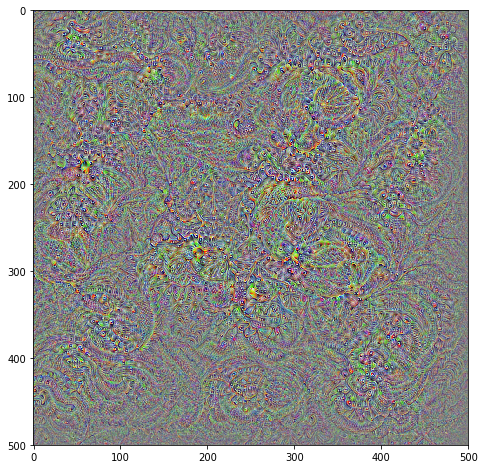# Visualising CNNs: The Class Appearance Model¶

In this example we will demonstrate the ClassAppearanceModel callback included in torchbearer. This implements one of the most simple (and therefore not always the most successful) deep visualisation techniques, discussed in the paper Deep Inside Convolutional Networks: Visualising Image Classification Models and Saliency Maps

Note: The easiest way to use this tutorial is as a colab notebook, which allows you to dive in with no setup. We recommend you enable a free GPU with

Runtime   →   Change runtime type   →   Hardware Accelerator: GPU

## Install Torchbearer¶

First we install torchbearer if needed.

In :
try:
import torchbearer
except:
!pip install -q torchbearer
import torchbearer

print(torchbearer.__version__)

0.4.0.dev


## Background¶

The process to obtain Figure 1 from the paper is simple, given a particular target class $c$, we use back-propagation to obtain

\begin{equation} \arg\!\max_I \; S_c(I) - \lambda\Vert I \Vert_2^2\; , \end{equation}

where $S_c(I)$ is the un-normalised score of $c$ for the image $I$ given by the network. The regularisation term $\Vert I \Vert_2^2$ is necessary to prevent the resultant image from becoming overly noisy. More recent visualisation techniques use much more advanced regularisers to obtain smoother, more realistic images.

Since we are just running the callback on a pre-trained model, we don't need to load any data in this example. Instead, we use torchvision to load a VGG16 trained on ImageNet with the following:

In :
import torch.nn as nn
import torchvision

class Model(nn.Module):
def __init__(self):
super(Model, self).__init__()
self.net = torchvision.models.vgg16(True)

def forward(self, input):
if input is not None:
return self.net(input)

model = Model()


We need to include the None check as we will initialise the Trial without a dataloader, and so it will pass None to the model forward.

## Running with the Callback¶

When producing gradient based visualisations, we commonly need to include a transform to convert the input to the right space. For torchvisions ImageNet models we can use the following:

In :
from torchvision import transforms

normalize = transforms.Normalize(
mean=[0.485, 0.456, 0.406],
std=[0.229, 0.224, 0.225]
)


Finally we can construct and run the Trial with:

In :
%matplotlib inline
import matplotlib
matplotlib.rcParams['figure.figsize'] = (8.0, 8.0)

import torch
from torchbearer import Trial
from torchbearer.callbacks import imaging

device = 'cuda' if torch.cuda.is_available() else 'cpu'

trial = Trial(model, callbacks=[
imaging.ClassAppearanceModel(1000, (3, 500, 500), steps=128, target=951, decay=0.002, in_transform=normalize)
.on_val().to_pyplot().to_file('lemon.png'),
imaging.ClassAppearanceModel(1000, (3, 500, 500), steps=128, target=968, decay=0.002, in_transform=normalize)
.on_val().to_pyplot().to_file('cup.png'),
imaging.ClassAppearanceModel(1000, (3, 500, 500), steps=128, target=543, decay=0.002, in_transform=normalize)
.on_val().to_pyplot().to_file('dumbbell.png')
])
trial.for_val_steps(1).to(device)
trial.evaluate()